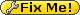fimex:todo:parameterfunction

# Project-Plan: easy function-based on demand parameter selection

currently moved to interal wiki for discussion https://dokit.met.no/personal/it/heikok/fimex_parameterfunction

Meteo. input data does not allways contain all parameters since gridded data usually is huge, while it is relatively easy to calculate them from other parameters, e.g. relative humidity ↔ specific humidity. An end user just want to have 'his' parameter, independently if this is stored on disk or calculated on demand. Fimex currently does not support an easily configurable interface to support calculated parameters.

Diana supports on demand parameters in the FieldCompute setup. But FieldCompute can only be used from within diana and is as such restricted to graphical users requirements, i.e. ~2s for display of data. And independent approach would avoid duplication and improve reliability if such on demand parameters could be added to all applications reading data via the fimex.

• The on demand parameters shall be usable from all programs which can read data via the libfimex interface, independently if it is C/C++, Fortran2003, R, the command-line or any future language.
• Users shall be able to change the order of functions, or the selected parameter for the on-demand function. (e.g. setup-files or strings in an input form)
• It shall be possible to have common on-demand rules, while it also must be possible to overrule or extend these common rules.
• On demand parameters must be recurse, i.e. one on demand parameter shall be able to rely on another on demand parameter.
• Users shall be able to switch on and off on demand parameters.
• It shall be possilbe to create an on-demand parameter from multiple CDMReader sources (as long as the data has the same grid)
• The system shall work with both variable-names and CF standard_names and automatically select the first / best.
• The system shall be aware of units, both on input-data, as well as when requested for output.
• The on demand parameters should be callable in the standard fimex way, i.e. extend a CDMReader, show an overview of all parameters, both real and on-demand and fetch the data by 'getDataSlice'.
• The software should have a clear separation from other fimex functionality, e.g. it should create a lib of its own, and it should reside in an own (sub-)catalog. It might be useful to integrate it into the fimex infrastruction, e.g. build / test infrastruction and even the same 'tar'-file (as long as the lib is a configure flag.)
• PI• plus
• minus
• multiply
• devide
• exp
• log
• ln• vertical interpolation? (10m, 80m)
• column (sum over levels)
• accumulation (time)
• deaccumulation (time)Diana field compute:

```<FIELD_COMPUTE>

# See diFieldFunctions.h or other documnetation

geopotential_height_pl=divide(geopotential_pl,9.81)

# computations in pressure levels (".plevel_")
air_temperature_pl=tk.plevel_th(air_potential_temperature_pl)
air_potential_temperature_pl=th.plevel_tk(air_temperature_pl:unit=kelvin)
the_pl=the.plevel_tk_rh(air_temperature_pl:unit=kelvin,relative_humidity_pl:unit=0.01)
the_pl=the.plevel_th_rh(air_potential_temperature_pl,relative_humidity_pl:unit=0.01)

thesat_pl=thesat.plevel_th(air_potential_temperature_pl)
thesat_pl=thesat.plevel_tk(air_temperature_pl:unit=kelvin)

relative_humidity_pl=rh.plevel_tk_q(air_temperature_pl:unit=kelvin,specific_humidity_pl:unit=0.01)
relative_humidity_pl=rh.plevel_th_q(air_potential_temperature_pl,specific_humidity_pl:unit=0.01)

dew_point_temperature_pl=tdc.plevel_th_q(air_potential_temperature_pl,specific_humidity_pl:unit=0.01)
dew_point_temperature_pl=tdc.plevel_tk_q(air_temperature_pl,specific_humidity_pl:unit=0.01)
dew_point_temperature_pl=tdc.plevel_th_rh(air_potential_temperature_pl,relative_humidity_pl:unit=0.01)
dew_point_temperature_pl=tdc.plevel_tk_rh(air_temperature_pl,relative_humidity_pl:unit=0.01)

specific_humidity_pl=q.plevel_tk_rh(air_temperature_pl:unit=kelvin,relative_humidity_pl:unit=0.01)
specific_humidity_pl=q.plevel_th_rh(air_potential_temperature_pl,relative_humidity_pl:unit=0.01)

qvx_pl=qvector.plevel_z_th_xcomp(geopotential_height_pl,air_potential_temperature_pl)
qvy_pl=qvector.plevel_z_th_ycomp(geopotential_height_pl,air_potential_temperature_pl)
qvx_pl=qvector.plevel_z_tk_xcomp(geopotential_height_pl,air_temperature_pl:unit=kelvin)
qvy_pl=qvector.plevel_z_tk_ycomp(geopotential_height_pl,air_temperature_pl:unit=kelvin)
qvectorx_pl=multiply(qvx_pl,1.e+11)
qvectory_pl=multiply(qvy_pl,1.e+11)
qvector.divergence_pl=divergence(qvectorx_pl,qvectory_pl)
ug_pl=geostrophic.wind.plevel_z_xcomp(geopotential_height_pl)
vg_pl=geostrophic.wind.plevel_z_ycomp(geopotential_height_pl)
uag_pl=subtract(x_wind_pl,ug_pl)
vag_pl=subtract(y_wind_pl,vg_pl)
</FIELD_COMPUTE>```
This website uses cookies. By using the website, you agree with storing cookies on your computer. Also you acknowledge that you have read and understand our Privacy Policy. If you do not agree leave the website.More information about cookies
• fimex/todo/parameterfunction.txt